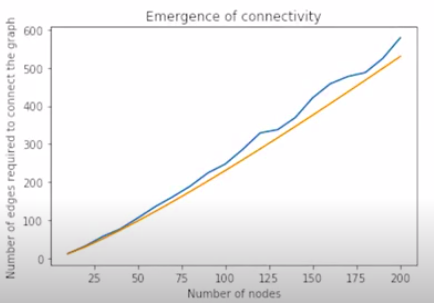GFG App
Open AppBrowser
Continue

# Emergence of connectedness in Social Networks

Prerequisite: Basics of NetworkX

The emergence of connectedness is to check whether the graph is connected or not. It says that in a graph of N nodes, we just need NLogN edges to make graph connected.

Approach:

The following algorithm to be followed:

1. Take any random graph with N number of nodes.
2. Now choose any node as a starting node.
3. Now add random edges to the graph and check every time if the graph is connected or not.
4. If the graph is not connected then repeat steps 2 and 3.
5. If the graph is connected then check the number of edges that are added and this will be equal to NLogN.
6. Now check both the plots will be almost similar.

Code for Checking connectedness:

## Python3

 `import` `networkx as nx ` `import` `matplotlib.pyplot as plt ` `import` `random ` ` `  `# Add N number of nodes in graph g  ` `# and return the graph ` `def` `add_nodes(N): ` `    ``g ``=` `nx.Graph() ` `    ``g.add_nodes_from(``range``(N)) ` `    ``return` `g ` ` `  `# Add 1 random edge ` `def` `add_random_edge(g): ` `    ``z1 ``=` `random.choice(g.nodes()) ` `    ``z2 ``=` `random.choice(g.nodes()) ` `    ``if` `z1 !``=` `z2: ` `        ``g.add_edge(z1, z2) ` `    ``return` `g ` ` `  `# Continue adding edges in graph g till  ` `# it becomes connected ` `def` `continue_add_connectivity(g): ` `    ``while``(nx.is_connected(g) ``=``=` `False``): ` `        ``g ``=` `add_random_edge(g) ` `    ``return` `g ` ` `  `# Creates an object of entire process. ` `# Input- number of nodes and Output-  ` `# number of edges required for graph ` `# connectivity. ` `def` `create_instance(N): ` `    ``g ``=` `add_nodes(N) ` `    ``g ``=` `continue_add_connectivity(g) ` `    ``return` `g.number_of_edges() ` ` `  `# Average it over 100 times ` `def` `create_average_instance(N): ` `    ``l ``=` `[] ` `    ``for` `i ``in` `range``(``0``, ``100``): ` `        ``l.append(create_instance(N)) ` `    ``return` `numpy.average(l) ` ` `  `# Plot the graph for different number  ` `# of edges ` `def` `plot_connectivity(): ` `    ``a ``=` `[] ` `    ``b ``=` `[] ` `     `  `    ``# j is the number of nodes ` `    ``j ``=` `10` `     `  `    ``while` `(j <``=` `1000``): ` `        ``a.append(j) ` `        ``b.append(create_average_instance(j)) ` `        ``i ``+``=` `10` `         `  `    ``plt.xlabel(``'Number of nodes'``) ` `    ``plt.ylabel(``'Number of edges required'``) ` `    ``plt.title(``'Emergence of connectivity'``) ` `    ``plt.plot(a, b) ` `    ``plt.show() ` ` `  `    ``a1 ``=` `[] ` `    ``b1 ``=` `[] ` `    ``j ``=` `10` `    ``while` `(j <``=` `400``): ` `        ``a1.append(j) ` `        ``b1.append(j``*``float``(numpy.log(j)``/``2``)) ` `        ``j ``+``=` `10` `    ``plt.plot(a1, b1) ` `    ``plt.show() ` ` `  ` `  `# main ` `plot_connectivity()`

Output:

```10
20
30
40
50
60
70
80
90
100
110
120
130
140
150
160
170
180
190
200
```Plot  of Emergence of Connectedness

My Personal Notes arrow_drop_up